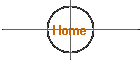### Elliptical Orbits and Kepler's Three Laws of Planetary Motion

Since the orbits of the planets are ellipses, let us review a few basic properties of ellipses.

1. For an ellipse there are two points called foci (singular: focus) such that the sum of the distances to the foci from any point on the ellipse is a constant. In terms of the diagram shown below, with "x" marking the location of the foci, we have the equation:

a + b = constantthat defines the ellipse in terms of the distances a and b.

2. The amount of "flattening" of the ellipse is termed the eccentricity. Thus, in the following figure the ellipses become more eccentric from left to right. A circle may be viewed as a special case of an ellipse with zero eccentricity, while as the ellipse becomes more flattened the eccentricity approaches one. Thus, all ellipses have eccentricities lying between zero and one.The orbits of the planets are ellipses but the eccentricities are so small for most of the planets that they look circular at first glance. For most of the planets one must measure the geometry carefully to determine that they are not circles, but ellipses of small eccentricity. Pluto and Mercury are exceptions: their orbits are sufficiently eccentric that they can be seen by inspection to not be circles.

3. The long axis of the ellipse is called the major axis, while the short axis is called the minor axis. Half of the major axis is termed a semi-major axis.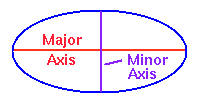The length of a semi-major axis is often termed the size of the ellipse. It can be shown that the average separation of a planet from the Sun as it goes around its elliptical orbit is equal to the length of the semi-major axis. Thus, by the "radius" of a planet's orbit one usually means the length of the semi-major axis.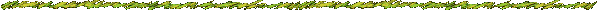## The Laws of Planetary Motion

Kepler obtained Brahe's data after his death despite the attempts by Brahe's family to keep the data from him in the hope of monetary gain. There is some evidence that Kepler obtained the data by less than legal means; it is fortunate for the development of modern astronomy that he was successful. Utilizing the voluminous and precise data of Brahe, Kepler was eventually able to build on the realization that the orbits of the planets were ellipses to formulate his Three Laws of Planetary Motion.

Kepler's First Law:

I. The orbits of the planets are ellipses, with the Sun at one focus of the ellipse.Kepler's First Law is illustrated in the image shown above. The Sun is not at the center of the ellipse, but is instead at one focus (generally there is nothing at the other focus of the ellipse). The planet then follows the ellipse in its orbit, which means that the Earth-Sun distance is constantly changing as the planet goes around its orbit. For purpose of illustration we have shown the orbit as rather eccentric; remember that the actual orbits are much less eccentric than this.

Why are the orbits elliptical and not circular? More about elliptical orbits

Kepler's Second Law:

II. The line joining the planet to the Sun sweeps out equal areas in equal times as the planet travels around the ellipse.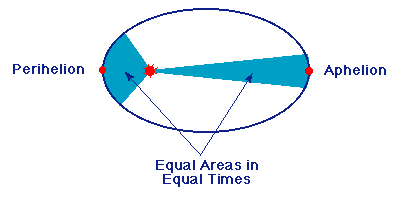Kepler's second law is illustrated in the preceding figure. The line joining the Sun and planet sweeps out equal areas in equal times, so the planet moves faster when it is nearer the Sun. Thus, a planet executes elliptical motion with constantly changing angular speed as it moves about its orbit. The point of nearest approach of the planet to the Sun is termed perihelion; the point of greatest separation is termed aphelion. Hence, by Kepler's second law, the planet moves fastest when it is near perihelion and slowest when it is near aphelion.

Kepler's Third Law:

III. The ratio of the squares of the revolutionary periods for two planets is equal to the ratio of the cubes of their semi-major axes.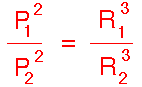In this equation P represents the period of revolution for a planet and R represents the length of its semi-major axis. The subscripts "1" and "2" distinguish quantities for planet 1 and 2 respectively. The periods for the two planets are assumed to be in the same time units and the lengths of the semi-major axes for the two planets are assumed to be in the same distance units.

Kepler's Third Law implies that the period for a planet to orbit the Sun increases rapidly with the radius of its orbit. Thus, we find that Mercury, the innermost planet, takes only 88 days to orbit the Sun but the outermost planet (Pluto) requires 248 years to do the same.

(For more detailed mathematical explanation, see this link.)

### Calculations Using Kepler's Third Law

A convenient unit of measurement for periods is in Earth years, and a convenient unit of measurement for distances is the average separation of the Earth from the Sun, which is termed an astronomical unit and is abbreviated as AU. If these units are used in Kepler's 3rd Law, the denominators in the preceding equation are numerically equal to unity and it may be written in the simple form

### P (years)2 = R (A.U.s)3

This equation may then be solved for the period P of the planet, given the length of the semi-major axis,

### P (years) = R (A.U.)3/2

or for the length of the semi-major axis, given the period of the planet,

### R (A.U.) = P (Years) 2/3

As an example of using Kepler's 3rd Law, let's calculate the "radius" of the orbit of Mars (that is, the length of the semi-major axis of the orbit) from the orbital period. The time for Mars to orbit the Sun is observed to be 1.88 Earth years. Thus, by Kepler's 3rd Law the length of the semi-major axis for the Martian orbit is

### R = P 2/3 = (1.88) 2/3 = 1.52 AU

which is exactly the measured average distance of Mars from the Sun. As a second example, let us calculate the orbital period for Pluto, given that its observed average separation from the Sun is 39.44 astronomical units. From Kepler's 3rd Law

### P = R3/2 = (39.44)3/2 = 248 Years

which is indeed the observed orbital period for the planet Pluto.

 10/01/2017